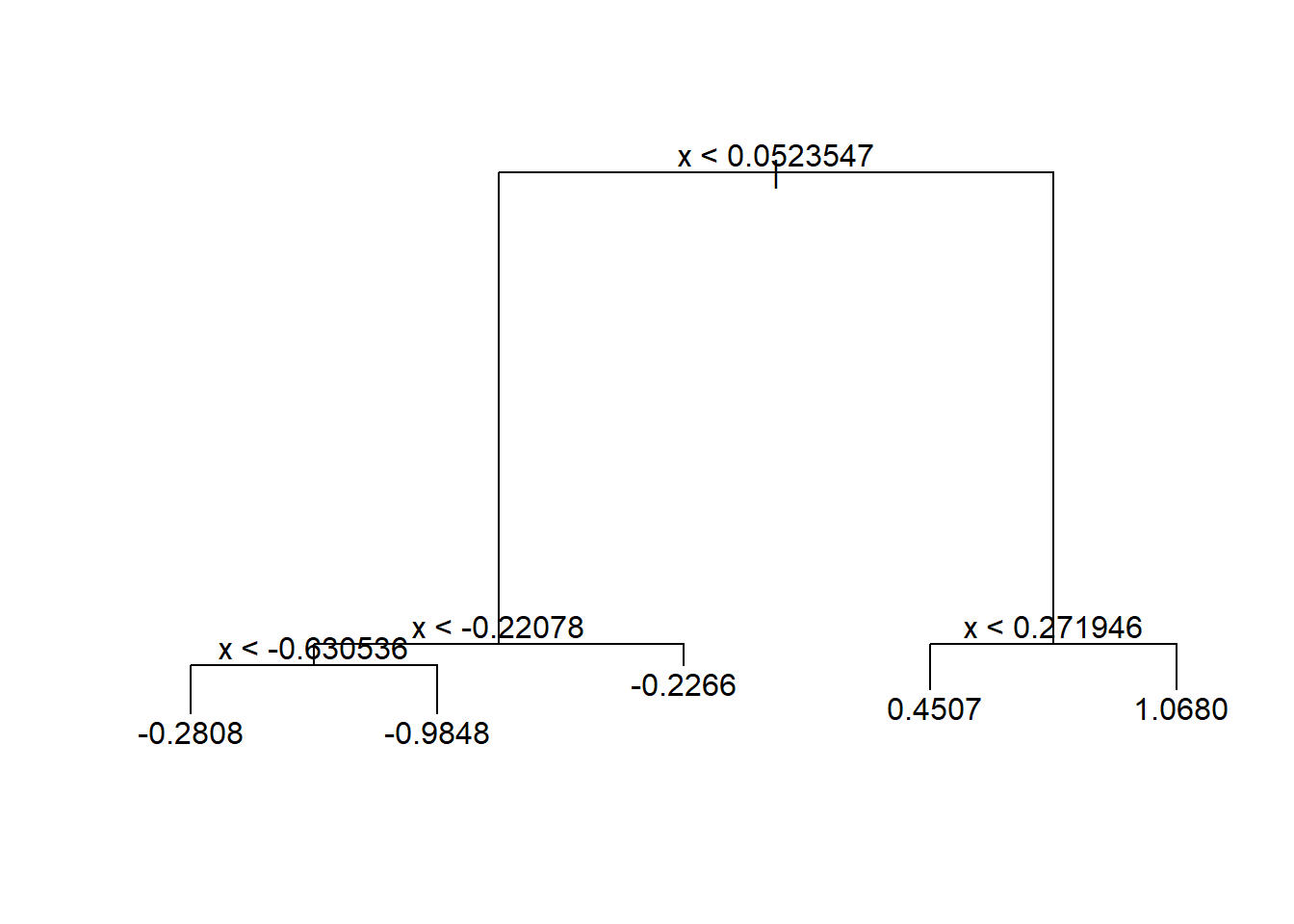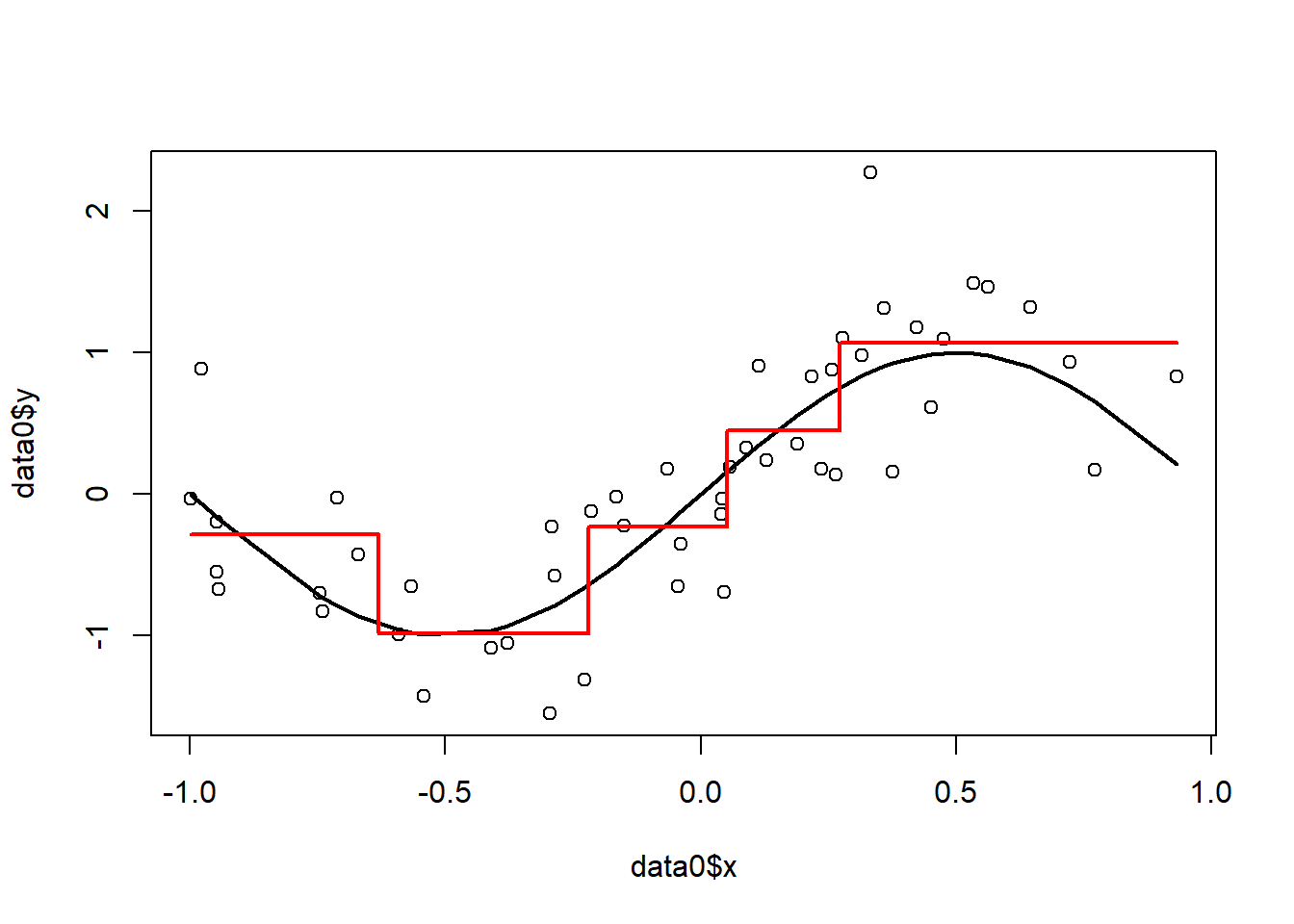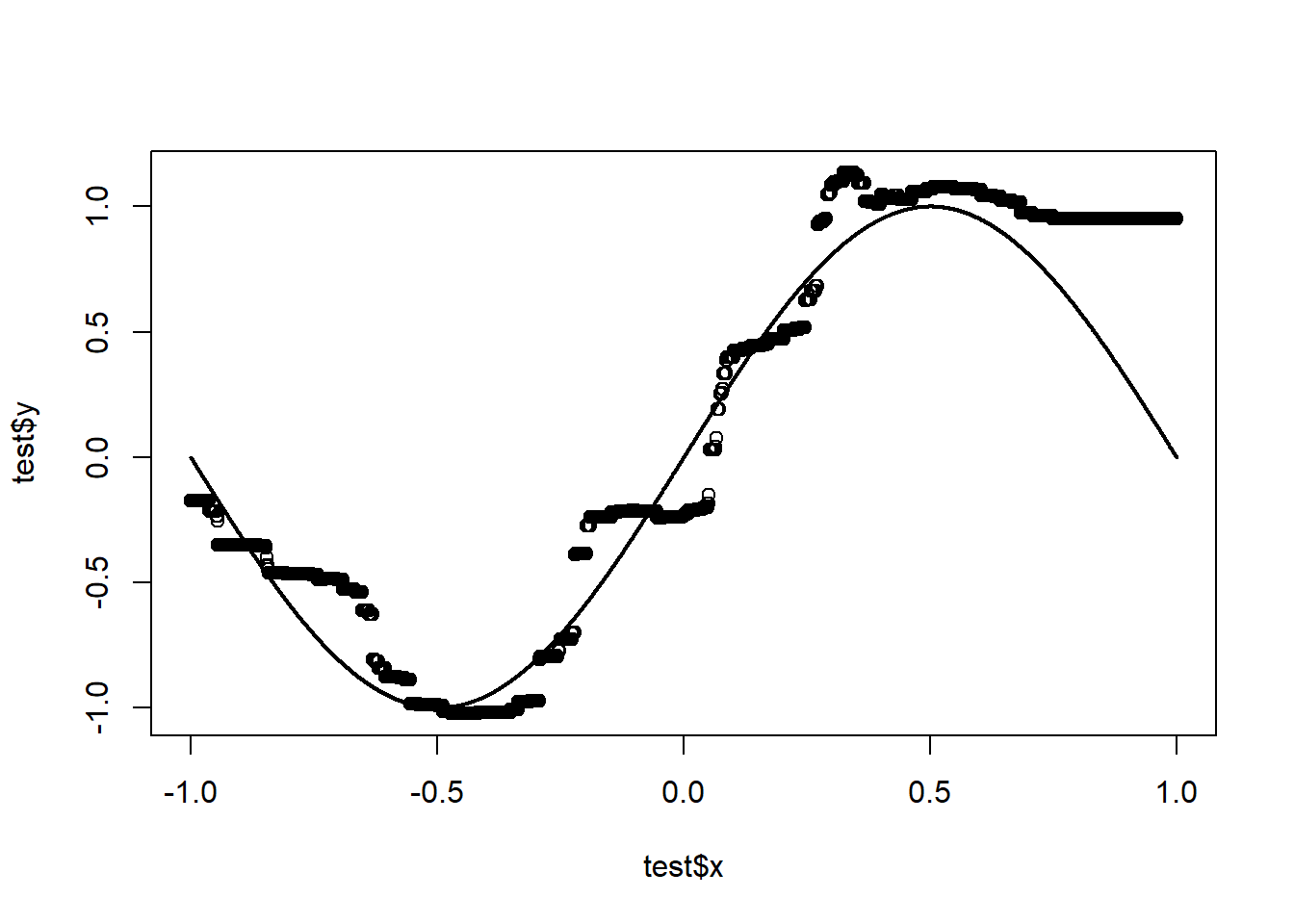### 1. Implement Bagging on the data generating model of Example 2.8 (use the file Example2.8_Bootstrap.html): (1) set parameter sigma=0.5, N=50; (2) build and visualize one tree model (you can use any software package) on one data sample; (3) manually generate 1000 bootstrap samples and build a bagging model, visualize the resulted prediction on test data, and measure the MSE, Bias, and Variance.

#### Using the data generating model of Example 2.8, as well as creating out of sample data, we have the following code.

#Data generating model
set.seed(135790)
# static parameters
x.min <- -1
x.max <- 1
sigma <- 0.5
# function for generating data
data.gen <- function(N=2) {
x <- runif(n=N, min=x.min, max=x.max)
f.x <- sin(pi * x)
epsilon <- rnorm(n=N, mean=0, sd=sigma)
y <- f.x + epsilon
return(data.frame(x=x, y=y))
}

#Out of Sample data
M <- 10000
x.out <- sort(runif(n=M, min=x.min, max=x.max))  # order the data points by x for easy plots
f.x.out <- sin(pi * x.out)
y.out <- f.x.out + rnorm(n=M, mean=0, sd=sigma)
x.test=data.frame(x=x.out)

#### Generating one data sample size N=50, sigma = 0.5

N=50;
data0 = data.gen(N=N);

#### Build one tree using ‘tree’ library.

#Buiding a tree
tree1 <- tree(y ~ x, data=data0)
summary(tree1)
##
## Regression tree:
## tree(formula = y ~ x, data = data0)
## Number of terminal nodes:  5
## Residual mean deviance:  0.2014 = 9.064 / 45
## Distribution of residuals:
##     Min.  1st Qu.   Median     Mean  3rd Qu.     Max.
## -0.91150 -0.26760 -0.03412  0.00000  0.25330  1.20400

#### and plot decision tree…

# plot the fitted tree
plot(tree1)
text(tree1)#### Plot the partitions

# plot the partition
plot(data0$x, data0$y)
x <- data0$x[order(data0$x)]
f.x <- sin(pi * x)
lines(x, f.x, lwd=2)
partition.tree(tree1, lwd=2, col="red", add=T)### bootstrapping

#### function for generating Bootstrap data sample

data.bootstrap <- function(data) {
index <- sample(N, N, replace=TRUE)
return(data[index, ])
}

#### generating 1000 trees and getting the predictions

RUN=1000;
predictions = matrix(rep(0,RUN*M),nrow = RUN, ncol=M)
#generate 1000 bootstrap samples and getting predictions
for (run in 1:RUN) {
data <- data.bootstrap(data = data0);
treeBS = tree(y~x,data=data);
predictions[run,] = predict(treeBS,x.test);
}
y.test = colMeans(predictions)

#### visualizing the non-random forest

test = data.frame(x=x.test, y=y.test)
plot(test$x, test$y);
lines(x.out, f.x.out, lwd=2);#### Measuring MSE, Bias, and Variance

g_D_x <- predictions;
g_bar_x <-colMeans(g_D_x)

bias = mean((g_bar_x-f.x.out)^2);
var = mean((t(g_D_x)-g_bar_x)^2);
MSE = mean((t(g_D_x)-y.out)^2);

The final numbers are as follows.

Bias Variance MSE
0.0676821 0.0616438 0.3769345

### 2. Show that as the number of bootstrap samples B gets large, the OOB error estimate for a random forest approaches its LOOCV error estimate, and that in the limit, the identity is exact.

We first prove that each bootstrap sample takes about $$2/3$$ of the original sample.

The probability of an observation $$(x_i,y_i)$$ not being selected from selecting from sample of size $$N$$ is $$(1-\frac{1}{N})$$. For a bootstrap sample of size $$N$$, the probability of not selecting $$(x_i,y_i)$$ is $$(1-\frac{1}{N})^N\rightarrow\frac{1}{e}\approx\frac{1}{3}$$ as $$N\rightarrow\infty$$. Hence, each bootstrap sample takes about $$1-\frac{1}{3}=\frac{2}{3}$$ of the observations.

Let $$X$$ be a sample of size $$N$$. Let $$X_t$$ be a sample drawn with repetition from $$X$$, used to build tree $$t$$, then $$X_t$$ is a bootstrap sample of $$X$$ for tree $$t$$. Let $$O_t = X \setminus X_t$$ be the set of out-of-bag elements of tree $$t$$. Then we have

\begin{align*} \text{OOB Error}&=\frac{\sum\limits_{t=1}^{B}\sum\limits_{i=1}^{|O_t|} Error(y_i,f_t(x_i))}{\sum\limits_{t=1}^{B}|O_t|} \end{align*}

To compute the LOOCV, we partition $$X$$ into $$N$$ partitions $$X=\{X_1,X_2,\dots,X_N\}$$, $$X_i\cap X_j=\phi$$, $$|X_i|=1$$ since the sample size is $$N$$. Let $$S_t=X\setminus{X_t}$$ be the sample used for creating the random forest $$t$$. Then we have

\begin{align*} \text{LOOCV error}=\frac{\sum\limits_{t=1}^{N}Error(y_t,f_{S_t}(x_t))}{N} \end{align*} We also proof that with probability 1 each element is selected in at least one of the bootstrap samples. The probability of an observation $$(x_i,y_i)$$ not being selected for a particular bootstrap sample is $$(1-\frac{1}{N})^N$$. Hence, for $$B$$ number of bootstrap samples, the probability of $$(x_i,y_i)$$ not being selected at all is $$(1-\frac{1}{N})^{NB}\rightarrow0$$ as $$B\rightarrow\infty$$. Now we are ready to show the convergence of the OOB Error. \begin{align*} \lim_{B\rightarrow\infty}\text{OOB Error}&=\frac{\lim_{B\rightarrow\infty}\sum\limits_{t=1}^{B}\sum\limits_{i=1}^{|O_t|} Error(y_i,f_t(x_i))}{\lim_{B\rightarrow\infty}\sum\limits_{t=1}^{B}|O_t|}\\ &=\frac{\frac{1}{3}\sum\limits_{i=1}^{N} Error(y_i,f_t(x_i))}{\frac{1}{3}N}\\ &=\frac{\sum\limits_{i=1}^{N} Error(y_i,f_t(x_i))}{N} \end{align*}